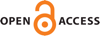Download this articleFor screen For printingRecent IssuesThe Journal About the Journal Editorial Board Editorial Interests Subscriptions Submission Guidelines Submission Page Policies for Authors Ethics Statement ISSN (electronic): 1472-2739 ISSN (print): 1472-2747 Author Index To Appear Other MSP JournalsDiffeomorphisms of odd-dimensional discs, glued into a manifold

### Johannes Ebert

Algebraic & Geometric Topology 23 (2023) 2329–2345##### Abstract

Let ${\mu }_{M}:{\mathrm{BDiff}}_{\partial }\left({D}^{2n+1}\right)\to \mathrm{BDiff}\left(M\right)$, for a compact $\left(2n+1\right)$–dimensional smooth manifold $M$, be the map defined by extending diffeomorphisms on an embedded disc by the identity. By a classical result of Farrell and Hsiang, the rational homotopy groups and the rational homology of ${\mathrm{BDiff}}_{\partial }\left({D}^{2n+1}\right)$ are known in the concordance stable range. We prove two results on the behaviour of the map ${\mu }_{M}$ in the concordance stable range. Firstly, it is injective on rational homotopy groups, and secondly, it is trivial on rational homology if $M$ contains sufficiently many embedded copies of ${S}^{n}×{S}^{n+1}\setminus \mathrm{int}\left({D}^{2n+1}\right)$. We also show that ${\mu }_{M}$ is generally not injective on homotopy groups outside the stable range.

The homotopical statement is probably not new and follows from the theory of smooth torsion invariants. The noninjectivity outside the stable range is based on recent work by Krannich and Randal-Williams. The homological statement relies on work by Botvinnik and Perlmutter on diffeomorphisms of odd-dimensional manifolds.

##### Keywords
diffeomorphism groups of high-dimensional manifolds, smooth torsion invariants
Primary: 57S05
##### Publication